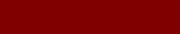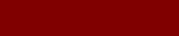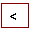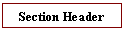The harder you work,            the luckier you seem to be.Spectral Analysis with gnu Octave
 The dispAnalysis() function demonstrates averaging the magnitudes of the basis functions over time (i.e. sampleSets) in order to minimize the effects of random “white noise” in the frequency spectrum.  For convenience, I decided to average these values for 1 second.  These values are then displayed as a semilog plot over the audio spectrum using the gnuPlot application (figure 2).               global yf1tot = zeros(size(yf1));              global yf2tot = zeros(size(yf2));              yf1tot = yf1tot .+ yf1;              yf2tot = yf2tot .+ yf2;              if (mod(sampleSet, 1/tdelta) > 0.005)                  return;              endif              yf1tot = yf1tot * tdelta;              yf2tot = yf2tot * tdelta;              The dispAnalyzer() function demonstrates an alternate method for minimizing the effects of random “white noise”.  It defines a set of audio bands, and calculates the average magnitude of all the basis functions that fall within each band.  These are displayed as bar graphs using gnuPlot (figure 3).              for ii=1:1:size(yf1,1)                  if ((ii * basisBW) > freqRanges(jj +1))                      jj = jj + 1;                  end                  ybin1(jj) = ybin1(jj) + yf1(ii);                  ybin2(jj) = ybin2(jj) + yf2(ii);                  nbin(jj)  = nbin(jj)  + 1;              end              ybin1 = ybin1./nbin;              ybin2 = ybin2./nbin;4  of  4www.MichaelDWelch.net                                                                                   MichaelDWelch@(no-spam)acm.org www.linkedIn.com/in/MichaelDWelch                                                                 303-920-9749      remove (no-spam)